Introduction to Chemical Kinetics: Rate, Rate Law & Order

# Introduction to Chemical Kinetics: Rate, Rate Law & Order Notes | Study Physical Chemistry - Chemistry

## Document Description: Introduction to Chemical Kinetics: Rate, Rate Law & Order for Chemistry 2022 is part of Physical Chemistry preparation. The notes and questions for Introduction to Chemical Kinetics: Rate, Rate Law & Order have been prepared according to the Chemistry exam syllabus. Information about Introduction to Chemical Kinetics: Rate, Rate Law & Order covers topics like Chemical Kinetics, Rate of Reaction, Rate Law and Rate Constant and Introduction to Chemical Kinetics: Rate, Rate Law & Order Example, for Chemistry 2022 Exam. Find important definitions, questions, notes, meanings, examples, exercises and tests below for Introduction to Chemical Kinetics: Rate, Rate Law & Order.

Introduction of Introduction to Chemical Kinetics: Rate, Rate Law & Order in English is available as part of our Physical Chemistry for Chemistry & Introduction to Chemical Kinetics: Rate, Rate Law & Order in Hindi for Physical Chemistry course. Download more important topics related with notes, lectures and mock test series for Chemistry Exam by signing up for free. Chemistry: Introduction to Chemical Kinetics: Rate, Rate Law & Order Notes | Study Physical Chemistry - Chemistry
 Table of contents Chemical Kinetics Rate of Reaction Rate Law and Rate Constant
 1 Crore+ students have signed up on EduRev. Have you?

Chemical Kinetics

“Chemical Kinetics involves the study of the rates and mechanism of chemical reactions.”

Rate of Reaction

The rate of reaction refers to the speed at which the products are formed from the reactants in a chemical reaction.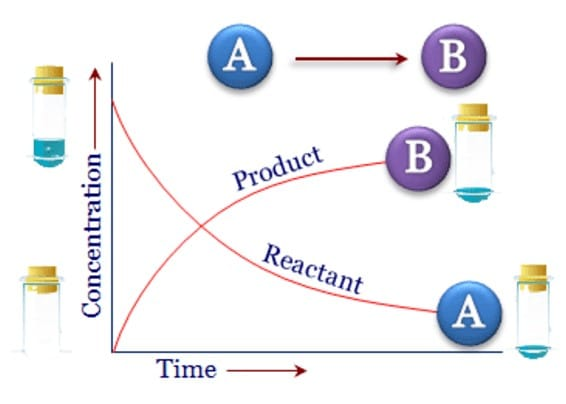Consider a reaction of the form
A + 2B  → 3C + D...........(1)
in which the molar concentration of participants are [A], [B], [C] & [D].
The rate of consumption or decomposition of one of the reactants at a given time is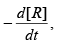where R is A or B. The rate of formation of one of the products is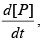where P is C or D.
The rate of reaction can be expressed with respect to any species in equation (1).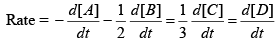Thus, the rate of reaction can be defined with respect to both reactants and products.
For example:
4NO2 (g) + O2 (g)  → 2N 2O2 (g)
Q.1. Find the expression for the Rate of Reaction.
4NO2 (g) + O2 (g)  → 2N 2O2 (g)

Sol: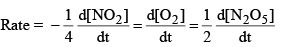Rate Law and Rate Constant

The rate of a reaction will generally depend on temperature pressure and the concentration of species involving in the reaction.
The rate of reaction is proportional to the molar concentration of reacting species.
i.e. A + B + C + D + ……. → Product
then, rate of reaction = k[A][B]b [C]c [D]d ………..

where [A] is the concentration of reactant A, [B] is the concentration of reactant B and so on. The constant a is known as the reaction order with respect to species A, b the reaction order with respect to species B, and so on.
The overall reaction order is equal to the sum of the individual reaction orders (a + b + c + d + ……..). Finally, the constant k is the rate constant for the reaction.
The rate constant is not only dependent on concentration but also on temperature & pressure.

This relationship is known as a rate law.

Order of the Reaction

A + B + C + ………  → Product
The rate law = v = k[A]a [B]b [C]c ……..
The order of reaction = a + b + c + ……
For example: if rate law = v = k[A]1/2 [B]
Then, it is half order in A, first-order in B, and three half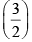order overall.

Molecularity of a Reaction

The number of reacting species (atoms, ions, or molecules) taking part in an elementary reaction, must collide simultaneously, in order to bring about a chemical reaction is called the molecularity of a reaction.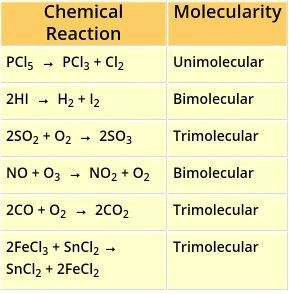Relationship between Rate law, Order, and the Rate Constant: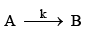Then, rate of reaction =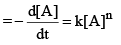The unit of rate of reaction is mol liter–1 sec–1 i.e. mol L–1 s–1.
where M represents mol L–1 or moles per liter & n is the order of reaction.
The unit of rate constant (k)
Rate of reaction = k[A]n
unit of rate of reaction = unit of k × [unit of concentration]
MS–1 = unit of k × [M]n

⇒  unit of k =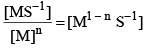i.e., unit of k     = M1 - n S-1 = mol1 - n Ln - 1 S-1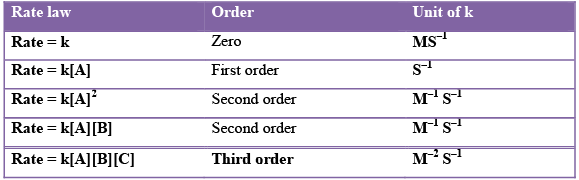Q.2. Find the order of the reaction if unit of rate constant or the reaction is (dm3)3/2 mol–3/2 s–1.
Sol.
Unit of rate constant = (dm3)3/2 mol–3/2 s–1 (given)
We know that,
Unit of rate constant = M1 – n s–1
For nth order
i.e. M1 - n s -1 = (dm3 )3 / 2 (mol)-3 / 2 s -1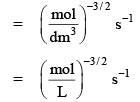∵      1 L = 1 dm3
&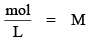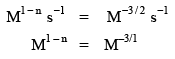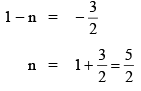i.e. it is 5/2 order reaction.
Determination of Order of Reaction

Using the following data for the reaction, we determine the order of the reaction with respect to A and B, over all order and rate constant for the reaction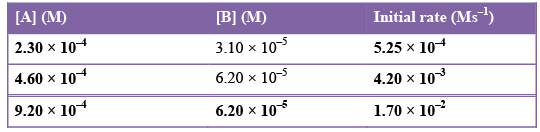Sol. A + B  → Product
rate of react ion = k[A]a [B]b
5.25 × 10–4 = k[2.30 × 10–4]a [3.10 × 10–5]b ...(1)
4.20 × 10–3 = k[4.60 × 10–4]a [6.20 × 10–5]...(2)
1.70 × 10–2 = k[9.20 × 10–4]a [6.20 × 10–5]b ...(3)

Divide equation (2) by equation (3), we get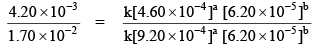2.47 × 10–1 = (0.5)a
(0.247) = (0.5)a
(0.5 × 0.5) ≈ (0.5)a
(0.5)a ≈ (0.5)a
or taking log we can find the value of a.
a = 2
Divide equation (1) by equation (2) we get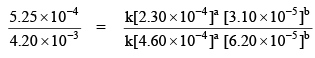1.25 × 10–1 = [0.5]a [0.5]b = [0.5]2 [0.5]b
= 0.25 [0.5]b
5 × 10–1 = [0.5]b
⇒ 0.5 = [0.5]b
⇒ b = 1

Therefore, the reaction is second order in A and first order in B, and third-order overall.

rate = k[A]2 [B]
5.2 × 10–4 Ms–1 = k(2.3 × 10–4 M)2 (3.1 × 10–5]M
⇒ k = 3.17 × 108 M–2 s–1
i.e. the over all rate law is
rate = (3.17 × 108 M–2 s–1) [A][B]

The document Introduction to Chemical Kinetics: Rate, Rate Law & Order Notes | Study Physical Chemistry - Chemistry is a part of the Chemistry Course Physical Chemistry.
All you need of Chemistry at this link: Chemistry

## Physical Chemistry

67 videos|106 docs|31 tests
 Use Code STAYHOME200 and get INR 200 additional OFF

## Physical Chemistry

67 videos|106 docs|31 tests

Track your progress, build streaks, highlight & save important lessons and more!

,

,

,

,

,

,

,

,

,

,

,

,

,

,

,

,

,

,

,

,

,

,

,

,

;